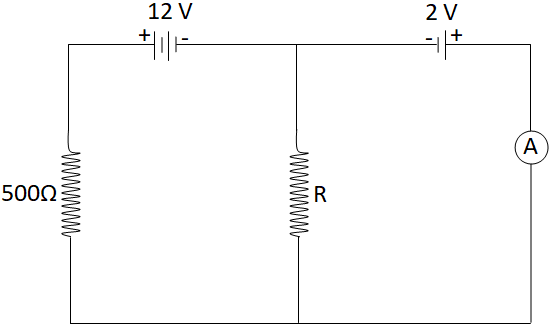QuestionAnswers

# In the given circuit shown below, the ammeter reading is zero. Then the value of the resistance $R$ is:Hint: In order to deal with this question first we will assume the current in the given circuit then we will proceed further by determining this current flowing through 500 ohm resistor further we apply ohm's law to get the value of unknown resistance.

Formula used- $V = IR$

Complete step-by-step solution -

Let's consider that , “i” is the current that passes through the circuit. In the specified circuit the reading of the ammeter is zero.
Hence, the voltage drop across $500\Omega$ resistance is $10V$ (as the batteries are oppositely connected).
As we know that according to the ohms law the relation between voltage, current and resistance is:
$V = IR \\ \Rightarrow I = \dfrac{V}{R} \\$
So, the current through $500\Omega$ resistance is,
$\because I = \dfrac{V}{R} \\ \Rightarrow {I_{500\Omega }} = \dfrac{{10V}}{{500\Omega }} \\ \Rightarrow {I_{500\Omega }} = \dfrac{1}{{50}}A \\$
As we know that the current through the ammeter path is zero, so this current flowing through $500\Omega$ resistor must all be flowing through the resistor “R”.
$\because {I_{500\Omega }} = \dfrac{1}{{50}}A \\ \Rightarrow {I_R} = \dfrac{1}{{50}}A \\$
And the voltage across the resistor “R” is 2V which is the same as the second cell.
As, now as we know the current and voltage across the resistor “R”; so, let us now use ohm’s law across the resistor “R” in order to find the value of resistance R.
$\because V = IR \\ \Rightarrow R = \dfrac{V}{I} \\ \Rightarrow R = \dfrac{{2V}}{{\left( {\dfrac{1}{{50}}A} \right)}} \\ \Rightarrow R = 50 \times 2\Omega \\ \Rightarrow R = 100\Omega \\$
Hence, the value of resistance is 100 ohm.

Note- Ohm's Law states that the current flowing through a conductor is directly proportional to the potential difference applied at its ends, provided the temperature and other physical conditions remain unchanged. Mathematically it can be represented as $V = IR$ . Ohm’s Law is used to determine the voltage, resistance or current of an electric circuit. Also it is used to maintain the desired voltage drop across the electronic components.
Integrated CircuitAC Voltage Applied to Series LCR CircuitCBSE Class 12 Physics Electric Charges and Fields FormulaElectric Current in ConductorsCBSE Class 12 Physics Nuclei FormulaCBSE Class 12 Physics Atoms FormulaProductivity in the EcosystemCBSE Class 12 Physics Electromagnetic Waves FormulaCBSE Class 12 Physics Electromagnetic Induction FormulaCBSE Class 12 Physics Wave Optics FormulaImportant Questions for CBSE Class 6 Social Science The Earth Our Habitat Chapter 1 - The Earth In The Solar SystemImportant Questions for CBSE Class 8 English Honeydew Chapter 1 - The Best Christmas Present in the WorldImportant Questions for CBSE Class 12 PhysicsImportant Questions for CBSE Class 12 Chemistry Chapter 1 - The Solid StateImportant Questions for CBSE Class 12 Physics Chapter 1 - Electric Charges and FieldsImportant Questions for CBSE Class 12 Physics Chapter 12 - AtomsImportant Questions for CBSE Class 6 English A Pact with The Sun Chapter 6 - The Monkey and the CrocodileImportant Questions for CBSE Class 12 Chemistry Chapter 7 - The p-Block ElementsImportant Questions for CBSE Class 12 Chemistry Chapter 8 - The d and f Block ElementsImportant Questions for CBSE Class 12 Macro Economics Chapter 5 - Government Budget and the EconomyCBSE Class 12 Physics Question Paper 2020Previous Year Question Paper for CBSE Class 12 PhysicsPrevious Year Question Paper for CBSE Class 12 Physics - 2015Previous Year Question Paper for CBSE Class 12 Physics - 2018Previous Year Question Paper for CBSE Class 12 Physics - 2014Previous Year Question Paper for CBSE Class 12 Physics - 2013Previous Year Question Paper for CBSE Class 12 Physics - 2019Previous Year Physics Question Paper for CBSE Class 12 - 2017Physics Question Paper for CBSE Class 12 - 2016 Set 1 CPrevious Year Question Paper for CBSE Class 12 Physics - 2016 Set 1 SLakhmir Singh Physics Class 10 Solutions Chapter 6 - The Human Eyes and The Colorful WorldNCERT Solutions for Class 12 Physics Chapter 12 Atoms in HindiHC Verma Class 12 Physics Part-2 Solutions for Chapter 32 - Electric Current in ConductorsNCERT Solutions for Class 12 Chemistry Chapter 1 The Solid State in HindiNCERT Solutions for Class 12 Physics Chapter 1 Electric Charges and Fields In HindiNCERT Solutions for Class 12 Physics Chapter 1NCERT Solutions for Class 12 Physics in HindiRS Aggarwal Class 12 Solutions Chapter-28 The PlaneNCERT Solutions for Class 12 Physics Chapter 15 Communication Systems in HindiNCERT Solutions for Class 12 Physics Chapter 2 Electrostatic Potential and Capacitance in Hindi